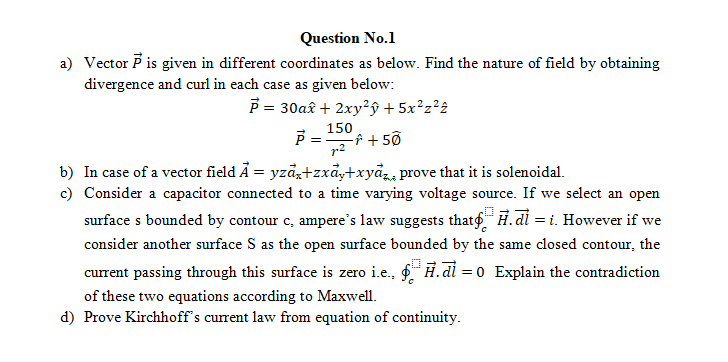# (Solved): Subject : Electromagnetic Field Theory please mention each step involving in the solution. a) Ve ...Subject : Electromagnetic Field Theory

please mention each step involving in the solution.

a) Vector $$\vec{P}$$ is given in different coordinates as below. Find the nature of field by obtaining divergence and curl in each case as given below: $\begin{array}{c} \vec{P}=30 a \hat{x}+2 x y^{2} \hat{y}+5 x^{2} z^{2} \hat{z} \\ \vec{P}=\frac{150}{r^{2}} \hat{r}+5 \widehat{\emptyset} \end{array}$ b) In case of a vector field $$\vec{A}=y z \vec{a}_{\mathrm{x}}+z x \vec{a}_{\mathrm{y}}+x y \vec{a}_{z, n}$$ prove that it is solenoidal. c) Consider a capacitor connected to a time varying voltage source. If we select an open surface s bounded by contour c, ampere's law suggests that $$\oint_{c} \vec{H} \cdot \overrightarrow{d l}=i$$. However if we consider another surface $$S$$ as the open surface bounded by the same closed contour, the current passing through this surface is zero i.e., $$\oint_{c} \vec{H} \cdot \overrightarrow{d l}=0$$ Explain the contradiction of these two equations according to Maxwell. d) Prove Kirchhoff's current law from equation of continuity.

We have an Answer from Expert